# Iteration Maths

Here we will learn about iteration, including how to find approximate solutions to equations using iterative methods and work with general iterative processes to set up and solve problems.

There are also iteration worksheets based on Edexcel, AQA and OCR exam questions, along with further guidance on where to go next if you’re still stuck.

## What is iteration?

Iteration is a repeated mathematical process that allows us to find approximate solutions for equations.

To do this we need to know the iterative formula and the starting value.

The iterative formula tells us how to find the next solution, which we label as x_{n+1}, given the current solution, which we label as x_{n}.

The iterative formula changes for every equation and using a different starting number can greatly affect the values of the solutions. We usually label the starting number as x_{0}.

For example,

The iterative formula to determine the next integer is

x_{n+1}=x_{n}+1.

If we know that x_{0}=3 and we want to calculate the value of x_{1}, we would substitute this into the iterative formula above with n=0 (as x_{n=0}=x_{0}=3) to get

\begin{aligned} x_{0+1}&=x_{0}+1\\\\ x_{1}&=3+1=4 \end{aligned}

So x_{1}=4.

This iterative formula will always find the next integer in a sequence for any value of n.

### What is iteration?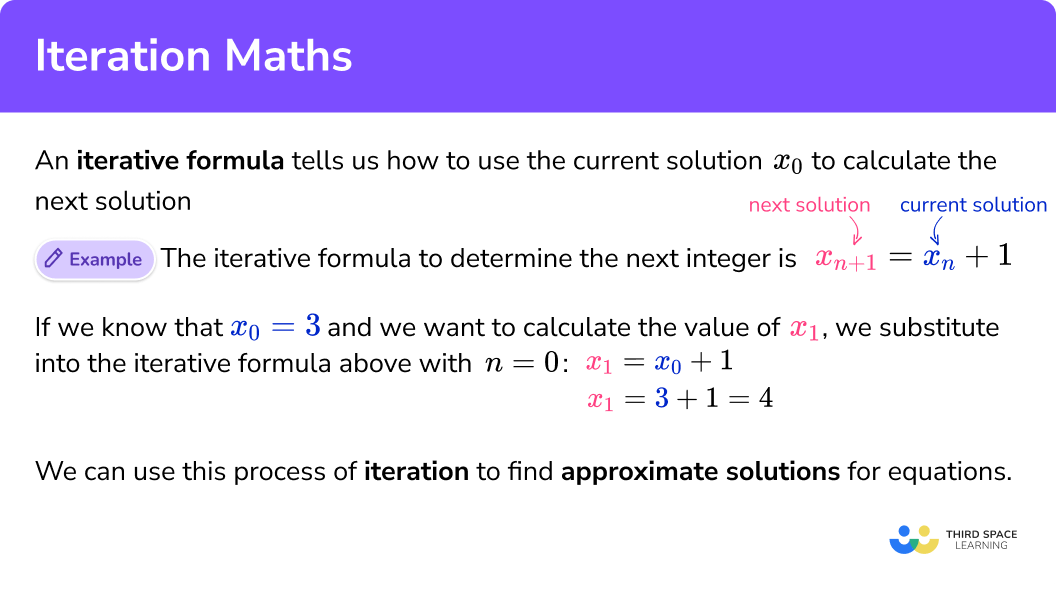### Iteration and sequences

Iteration generates a sequence of numbers.

• If the sequence tends towards a specific number (which is the approximate solution) then this is known as a converging sequence (or convergent sequence).

For example,

x_{n+1}=\frac{x_{n}}{10} converges towards 0 when x_{n} 0.

This is because each subsequent solution is the previous solution divided by 10 and so each number in the sequence would be 10 times smaller than the previous value.

However, if x_{n}=0 then we get a sequence of zeros as we repeat the calculation 0\div{10}=0.

• If the sequence does not tend towards a specific number, moreover the values become much more extreme, this is known as a diverging sequence (or divergent sequence).

For example,

x_{n+1}=3x_{n} is a diverging sequence when x_{n} 0.

This is because each subsequent solution is three times larger than the previous solution but if x_{n}=0 then we get a sequence of zeros as we repeat the calculation 3\times{0}=0.

• If the iteration cycles between one or more numbers, this is known as an oscillating sequence.

For example,

x_{n+1}=\frac{1}{x_{n}} oscillates between two numbers or is always equal to 1.

This is because, if we are continuously calculating the reciprocal of the previous value, the reciprocal of the reciprocal of a number is the original value (the reciprocal of 2 is \frac{1}{2}, and the reciprocal of \frac{1}{2} is 2 ) and so if x_{0}=2, we would generate the iterative sequence.

2, \ \frac{1}{2}, \ 2, \ \frac{1}{2}, \ 2, \ \frac{1}{2}, \ 2, \ \frac{1}{2},\ldots

If x_{0}=1, the reciprocal of 1 is 1 and so we would get the sequence 1, 1, 1, 1, 1,\ldots.

When we are finding an approximate solution to an equation using iteration, we are looking for a converging sequence. We can only approximate one solution using the value of x_{n}. To approximate another would require a different value for x_{n}.

Also, it is not always the case that when x_{n}=0 the sequence of values for the solutions oscillate. This is due to the specific nature of the iterative formula.

Solutions may be irrational numbers. Make sure that you do not round any value you are calculating with, only round your solution. For this reason, you may need to use a calculator to complete the iteration.

## How to use iteration

In order to use iteration to find an approximate solution to an equation:

1. Substitute x_{n} into the iteration formula.
2. Calculate the solution for x_{n+1} .
3. Repeat by substituting x_{n+1} into the iteration formula until the required approximation is reached.

### Explain how to use iteration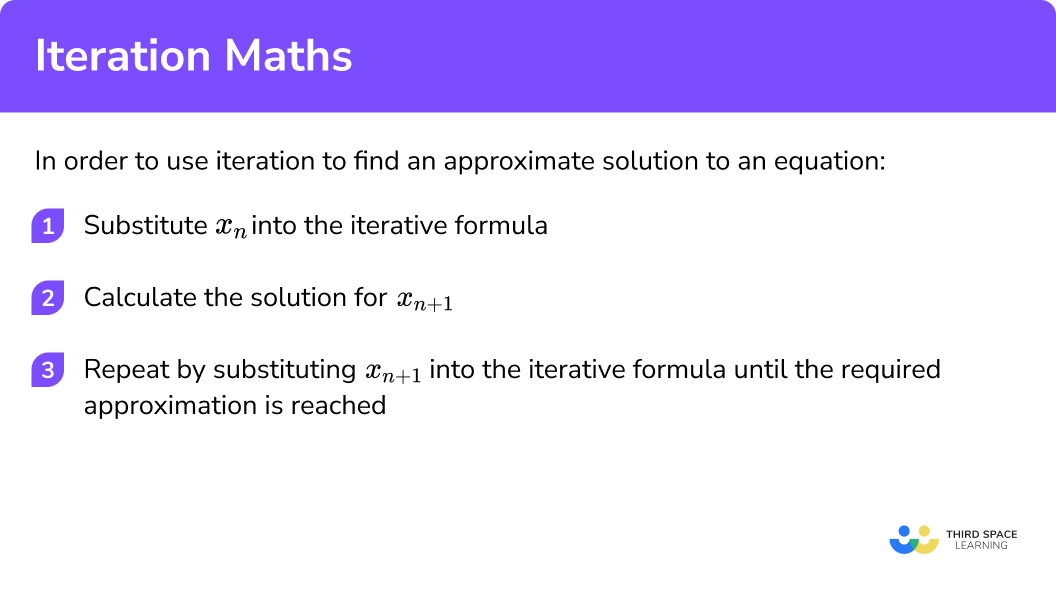### Related lessons on solving equations

Iteration maths is part of our series of lessons to support revision on solving equations. You may find it helpful to start with the main solving equations lesson for a summary of what to expect, or use the step by step guides below for further detail on individual topics. Other lessons in this series include:

## Iteration maths examples

### Example 1: iteration (diverging sequence)

Calculate the value of x_{3} for the iterative formula x_{n+1}=x_{n}+2 with x_{0}=1.

1. Substitute x_{n} into the iteration formula.

To calculate the value of x_{3}, we need to calculate the value of x_{1} and x_{2} first. As x_{0}=1, this means that n=0 and so substituting these values into the iteration formula, we have

\begin{aligned} x_{0+1}&=x_{0}+2\\\\ x_{1}&=1+2 \end{aligned}

2Calculate the solution for x_{n+1} .

Calculating the value of x_{1} we now have x_{1}=3.

3Repeat by substituting x_{n+1} into the iteration formula until the required approximation is reached.

Substituting x_{1}=3 into the iteration formula (now n=1 ), we have

\begin{aligned} x_{1+1}&=x_{1}+2\\\\ x_{2}&=3+2\\\\ x_{2}&=5 \end{aligned}

Substituting x_{2}=5 into the iteration formula (now n=2 ), we have

\begin{aligned} x_{2+1}&=x_{2}+2\\\\ x_{3}&=5+2\\\\ x_{3}&=7 \end{aligned}

The solution is x_{3}=7.

### Example 2: iteration (converging sequence)

Calculate the value of x_{2} for the iterative formula x_{n+1}=\frac{x_{n}}{6} with x_{0}=1296.

Substitute x_{n} into the iteration formula.

Calculate the solution for x_{n+1} .

Repeat by substituting x_{n+1} into the iteration formula until the required approximation is reached.

### Example 3: iteration (negative value of x₀)

Calculate the value of x_{3} for the iterative formula x_{n+1}=3x_{n}-5 with x_{0}=-2.

Substitute x_{n} into the iteration formula.

Calculate the solution for x_{n+1} .

Repeat by substituting x_{n+1} into the iteration formula until the required approximation is reached.

### Example 4: iteration including fractions

Calculate the value of x_{1}, x_{2} and x_{3} when x_{n+1}=\frac{7}{x_{n}}+4 and initial value x_{0}=4.

Substitute x_{n} into the iteration formula.

Calculate the solution for x_{n+1} .

Repeat by substituting x_{n+1} into the iteration formula until the required approximation is reached.

### Example 5: iteration including powers

Calculate the value of x_{3} when x_{n+1}=3x_{n}^{2} and x_{0}=\frac{1}{6}. Write your answer in standard form to a suitable degree of accuracy.

Substitute x_{n} into the iteration formula.

Calculate the solution for x_{n+1} .

Repeat by substituting x_{n+1} into the iteration formula until the required approximation is reached.

### Example 6: iteration including powers and fractions

Calculate the value of x_{2} when x_{n+1}=\frac{2}{x_{n}^{2}}-8 and x_{0}=0.4. Write your answer to 3 decimal places.

Substitute x_{n} into the iteration formula.

Calculate the solution for x_{n+1} .

Repeat by substituting x_{n+1} into the iteration formula until the required approximation is reached.

## Generating the iteration formula for an equation

To generate the iteration formula for an equation, we need to be able to rearrange the equation so that the subject x is also dependent on itself.

For example,

To explain the relationship between the quadratic equation x^{2}+8x-20=0 and the iterative formula x_{n+1}=\frac{20}{x_{n}}-8 we need to remove the labelling of x_{n+1} and x_{n} and rearrange this to get the quadratic.

Removing the labelling, we have

x=\frac{20}{x}-8.

Multiplying both sides of the equation by x we have

x^{2}=20-8x.

Adding 8x to both sides of the equation, we have

x^{2}+8x=20.

Finally subtracting 20 from both sides of the equation, we have

x^{2}+8x-20=0.

The iteration formula for an equation is a rearrangement of the equation, but we need to make sure that the variable x occurs on both sides of the equals sign.

## How to determine an iteration formula

In order to determine an iteration formula for an equation:

1. Rearrange the equation so that the highest power of x is the subject.
2. Divide throughout by one less than the highest power of x .
3. Rewrite using x_n and x_{n+1} .

### Explain how to determine an iteration formula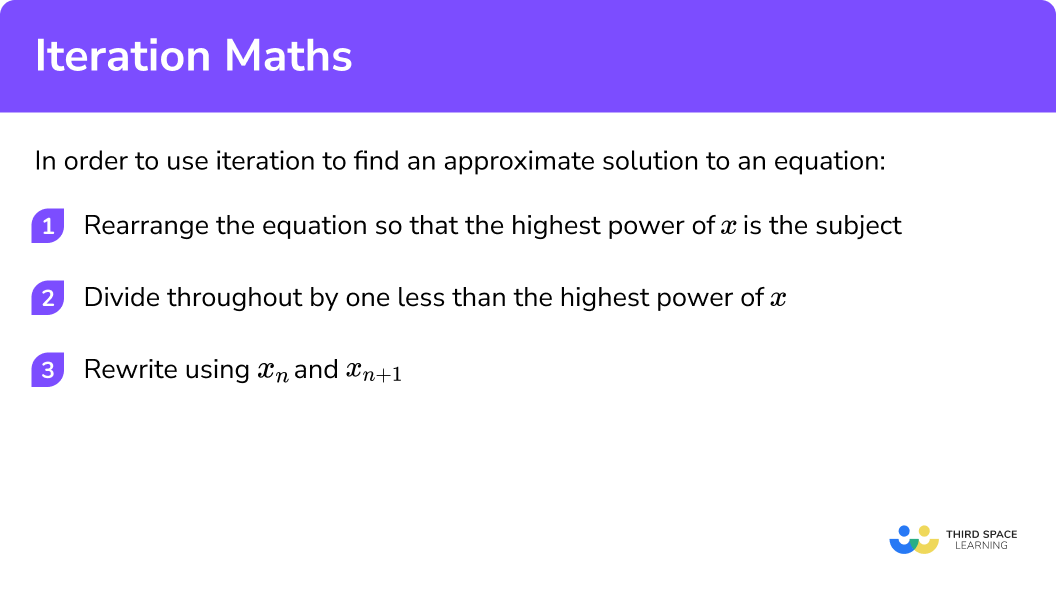### Example 7: generating an iterative formula

Determine an iterative formula that will calculate an approximate solution for the equation x^{3}-6x=0.

Rearrange the equation so that the highest power of x is the subject.

Divide throughout by one less than the highest power of x .

Rewrite using x_n and x_{n+1} .

### Common misconceptions

• Rounding too early

If you round the value for x_1 this will have an impact on the value of x_2. It is therefore important to only round the solutions, and not use rounded numbers within any further calculation.

• Incorrect labelling of solutions

When n=0 for x_{n}, the value of x_{n+1}=x_{0+1}=x_1 which is the next value in the sequence. This means that if we know the value of x_{0}, we can determine the value of x_1 (the next approximate solution).

• Calculating the final approximate solution straight away

If you are given x_0 and you need to calculate the value of x_3 , students would incorrectly try to calculate x_3 straight away rather than calculating the values of x_1 and x_2 first. We can only calculate the next term in the sequence if we know the current term and the iterative formula.

• x_{n+1} is the same as x_{n}+1

The algebraic notation is misinterpreted as 1 more than the value of x_{n} whereas the label n+1 is the next value in the sequence. Moreover, n is the current value in the sequence, and n-1 would be the previous value in the sequence.

### Practice iteration maths questions

1. Given the iterative formula x_{n+1}=x_{n}-3, calculate the value of x_{3} when x_{0}=12.

x_{3}=0x_{3}=3x_{3}=6x_{3}=9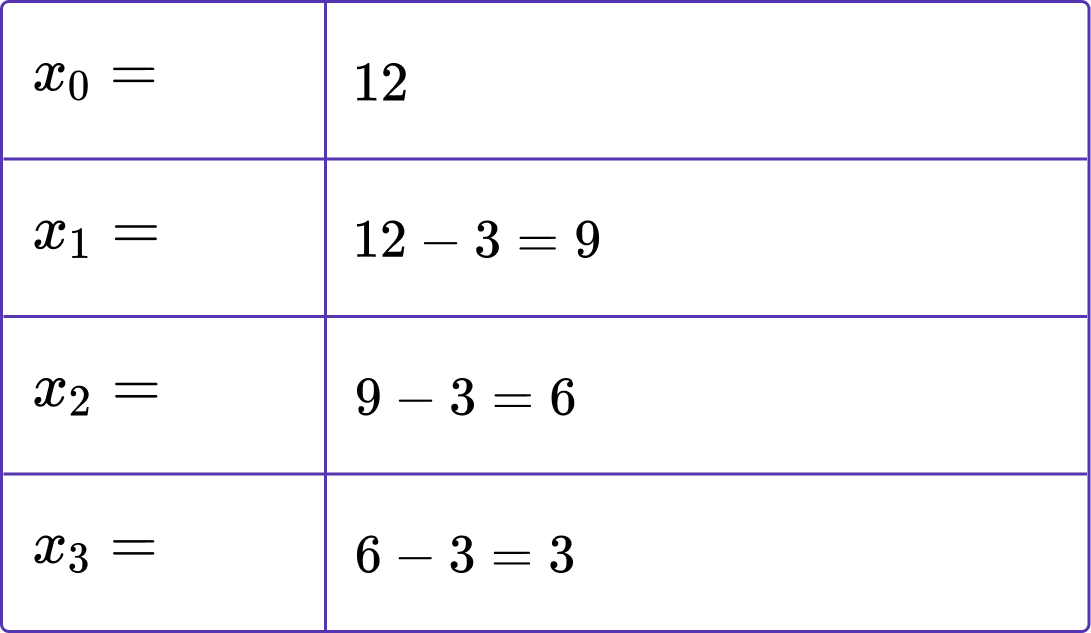2. Calculate the value of x_{2} when x_{0}=-1 and x_{n+1}=\frac{2}{5}x_{n}.

x_{2}=-0.16x_{2}=-0.4x_{2}=-1x_{2}=-2.5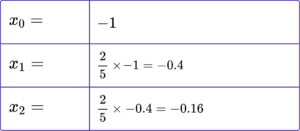3. Determine the value of x_{4} when x_{n+1}=3-2x_{n} and x_{0}=0.5.

x_{4}=-1x_{4}=2x_{4}=-7x_{4}=17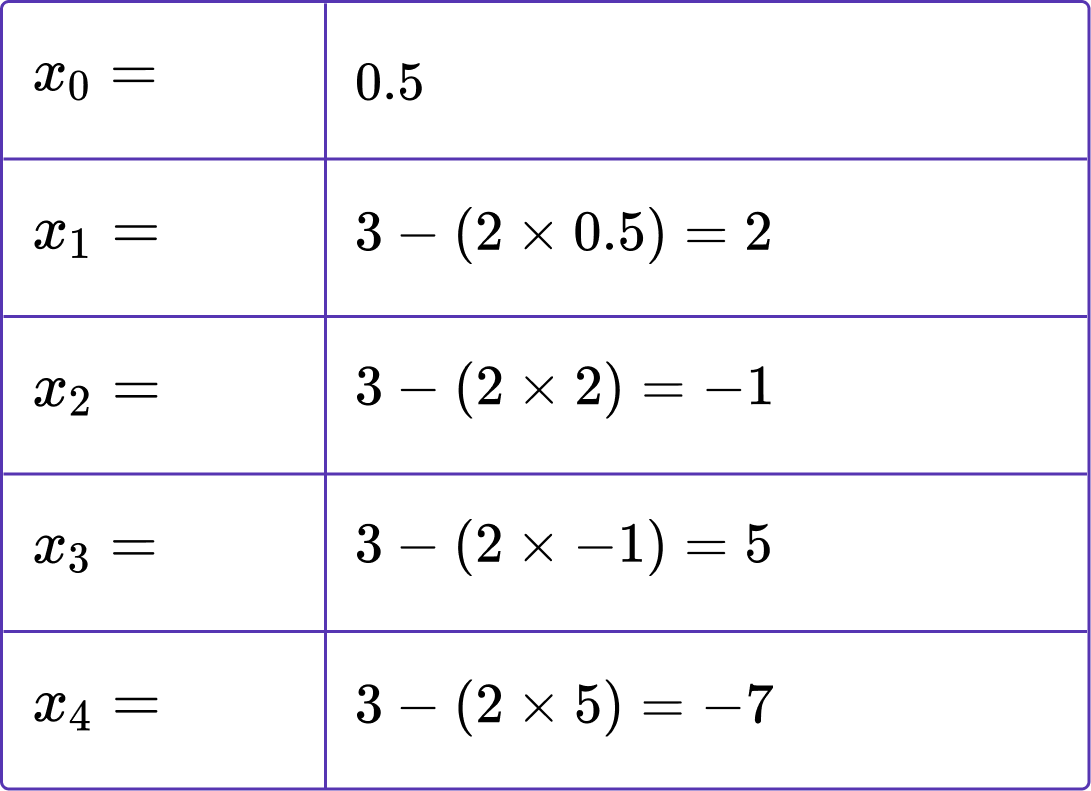4. Given that x_{0}=16 for the iterative formula x_{n+1}=5+\frac{4}{x_{n}}, calculate the value of x_{2} correct to 3 decimal places.

x_{2}=5.250 \ (3dp)x_{2}=5.694 \ (3dp)x_{2}=5.702 \ (3dp)x_{2}=5.762 \ (3dp)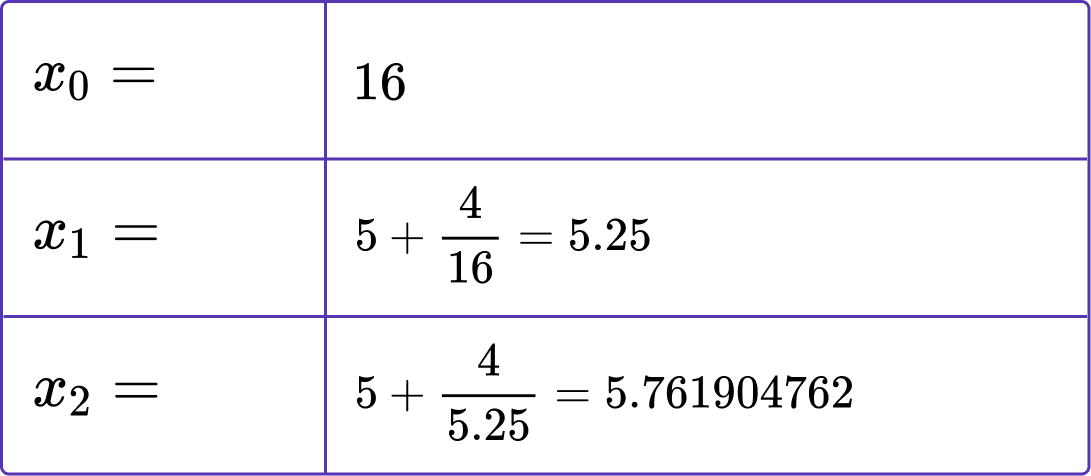5. Calculate the value of x_{3} when x_{0}=4 and x_{n+1}=\frac{x_{n}^{2}}{7}.

x_{3}=2.2857 \ (4dp)x_{3}=0.7464 \ (4dp)x_{3}=0.0796 \ (4dp)x_{3}=0.0009 \ (4dp)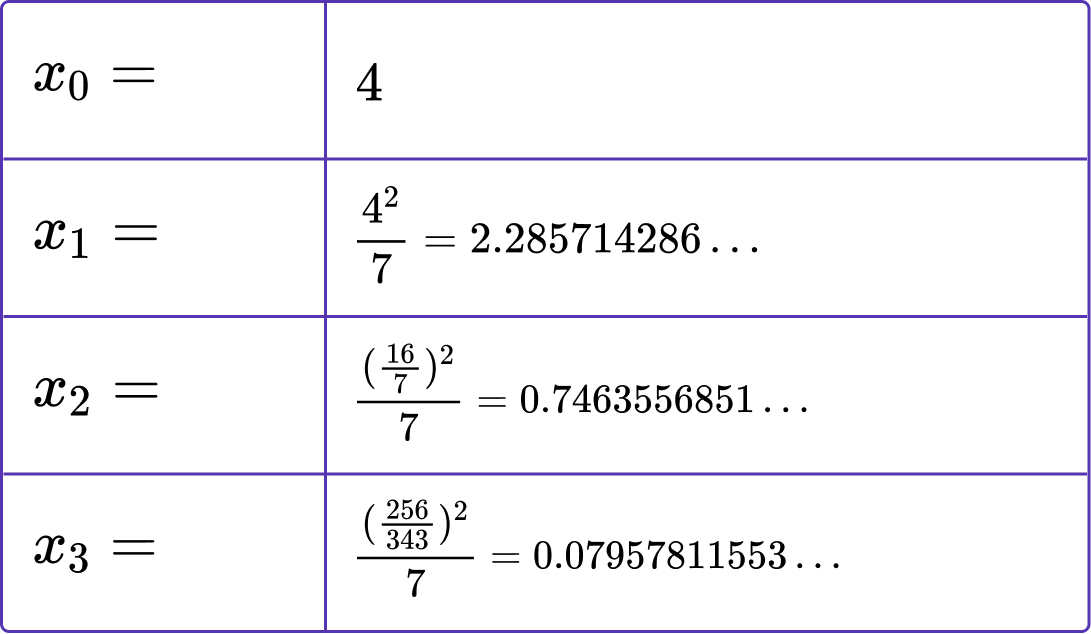6. Given that x_{n+1}=3-\frac{5}{x_{n}^{2}} and x_{1}=-2, calculate the value of x_{4}.

x_{4}=-44.1 \ (3dp)x_{2}=-2.000 \ (3dp)x_{4}=0.326 \ (3dp)x_{4}=1.367 \ (3dp)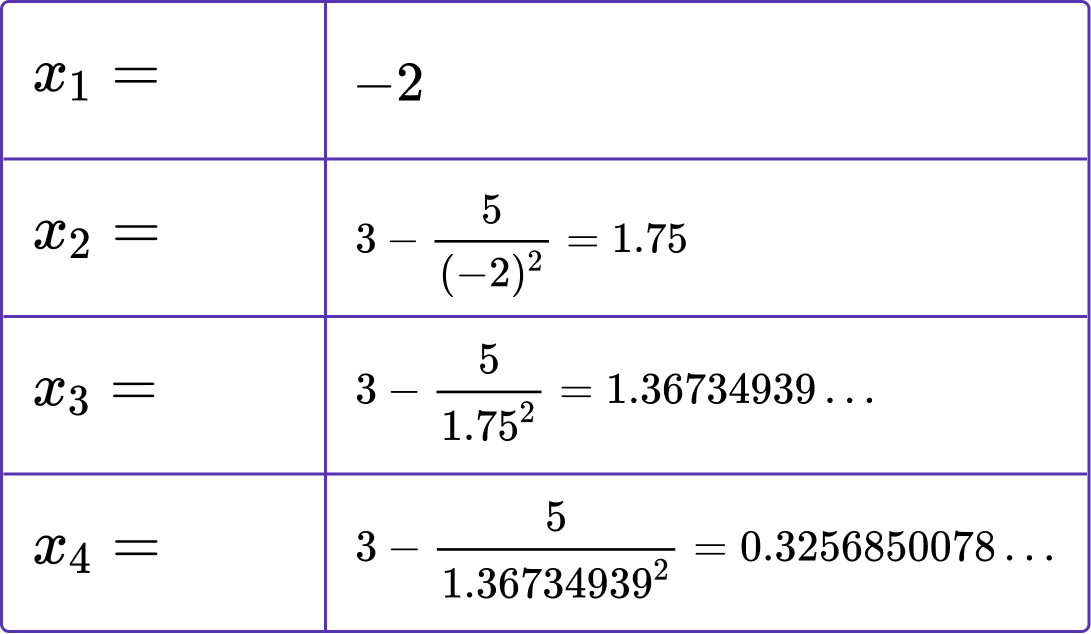7. Determine an iterative formula that would find an approximate solution for the equation \sqrt{x}-10x=4.

x_{n+1}=\frac{\sqrt{x_{n}}-4}{10}x_{n+1}=\frac{\sqrt{x_{n}}-10}{4}x_{n+1}=16+10x_{n}^{2}x_{n+1}=\frac{\sqrt{x_{n}+4}}{10}\begin{aligned} \sqrt{x}-10x&=4\\\\ \sqrt{x}&=4+10x\\\\ \sqrt{x}-4&=10x\\\\ \frac{\sqrt{x}-4}{10}&=x\\\\ x_{n+1}&=\frac{\sqrt{x_{n}}-4}{10} \end{aligned}

### Iteration maths GCSE questions

1. (a) Starting with x_{0}=1 and x_{1}=1, use the iteration formula x_{n+1}=x_{n-1}+x_n to determine the values of x_{2}, \ x_{3}, and x_{4}.

(b) Name the type of sequence generated by the iterative formula in part (a).

(4 marks)

(a)

x_{2}=1+1=2

(1)

x_{3}=1+2=3

(1)

x_{4}=2+3=5

(1)

(b)

Fibonacci Sequence

(1)

2. (a) Show that x^{2}+5x-6=0 can be rearranged to give x=\frac{6-x^2}{5}.

(b) Starting with x_{0}=0 use the iterative formula to find x_{3}.

(c) Describe the relationship between x_n and x^2+5x-6=0.

(7 marks)

(a)

x_{2}+5x=6

(1)

5x=6-x^2

(1)

x=\frac{6-x^2}{5} with division by 5 shown.

(1)

(b)

x_{1}=\frac{6-0^2}{5}=1.2

(1)

x_{2}=\frac{6-1.2^2}{5}=0.912

(1)

x_{3}=\frac{6-0.912^2}{5}=1.0336512

(1)

(c)

x_n is an approximate solution of the equation x^{2}+5x-6=0.

(1)

3. (a) Rearrange x^{2}+1=3x to get x=\sqrt{3x-1}.

(b) Given that x_{0}=3, use iteration to find the root of the equation x^{2}+1=3x correct to 1 decimal place.

(6 marks)

(a)

x^{2}=3x-1

(1)

x=\sqrt{3x-1} with the square root of both sides shown.

(1)

(b)

x_{n+1}=\sqrt{3x_{n}-1}

(1)

x_{1}=\sqrt{(3 \times 3)-1}=2.828427125

(1)

\begin{aligned} &x_{2}=\sqrt{(3 \times 2.828427125)-1}=2.735924227 \\ &x_{3}=\sqrt{(3 \times 2.735924227)-1}=2.684729536 \\ &x_{4}=\sqrt{(3 \times 2.684729536)-1}=2.655972253 \\ &x_{5}=\sqrt{(3 \times 2.655972253)-1}=2.639681185 \\ \end{aligned}

x_{6}=\sqrt{(3 \times 2.639681185)-1}=2.630407488 and minimum of 2 values of x_{n} above.

(1)

2.6 \ (1dp).

(1)

## Learning checklist

You have now learned how to:

• Find approximate solutions to equations numerically using iteration

## Still stuck?

Prepare your KS4 students for maths GCSEs success with Third Space Learning. Weekly online one to one GCSE maths revision lessons delivered by expert maths tutors.

Find out more about our GCSE maths tuition programme.